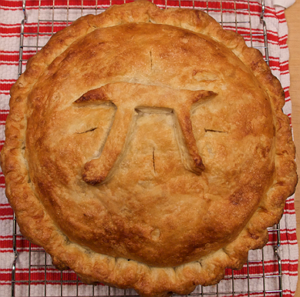# This problem is popular among the math lore

Algebra Level 5Today, I went to my grandmother's house to eat some delicious apple pie. However, being mischievous as always, she decided to test me with a question before letting me eat the pie.

$\large \lfloor x \lfloor x \lfloor x \lfloor x \rfloor\rfloor\rfloor\rfloor = 88$

If $\frac AB$ is the smallest positive value of $x$ that satisfy the equation above for coprime positive integer $A$ and $B$, what is the value of $A+B$?

###### Image Credit: Flickr Dennis Wilkinson.
×

Problem Loading...

Note Loading...

Set Loading...